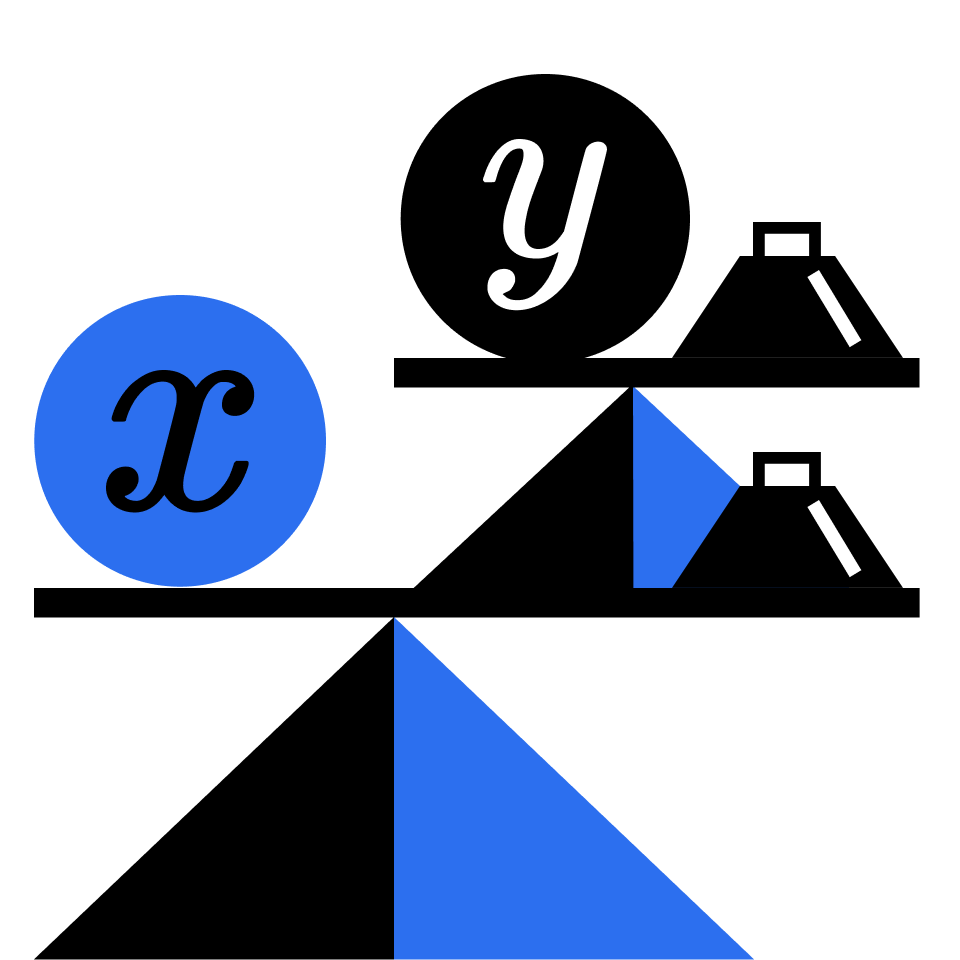# Algebra Fundamentals

Supercharge your algebraic intuition and problem solving skills!

\$0

38 Quizzes

### Course DetailsCourse Overview:

Explore how algebra works and why it matters, and build a strong foundation of skills across many algebra topics including equations, rates, ratios, and sequences.

By the end of this course, you’ll know both traditional algebraic techniques and many unique problem-solving approaches that aren’t typically covered in school. You'll also improve your algebraic intuition and hone your strategic thinking for approaching difficult problems.

### Topics covered:

• Arithmetic Sequences
• Algebra Shortcuts
• Balanced Scale Puzzles
• Calcdoku Puzzles
• Difference of Squares
• Fibonacci Numbers
• Geometric Sequences
• Inverse Square Laws
• Joint Proportionality
• Linear Equations
• Magic Squares
• Solving Systems of Equations
• ### Online Branch

Online, Online, Online

## Check out more ONLINE Math for Kids courses

### Pre Algebra 1

Arithmetic review, and move on to the distributive property, order of precedence, fractions and ratios. speed and rates of variationdecimals, percentages, negative numbersvariables and simplification of expressions.

by
• Price
• Duration

### Math and Computer Science

MIT graduate teaching math. Focused on geometry, algebra, trigonometry and calculus. Passionate about empowering the next generation.

by
• Price
• Duration
• Price
• Duration

### Early Elementary A: Discovering Numbers, Operations, and Measurement

This is an elementary-level course designed with 2nd grade math standards in mind.

by
• Price
• Duration